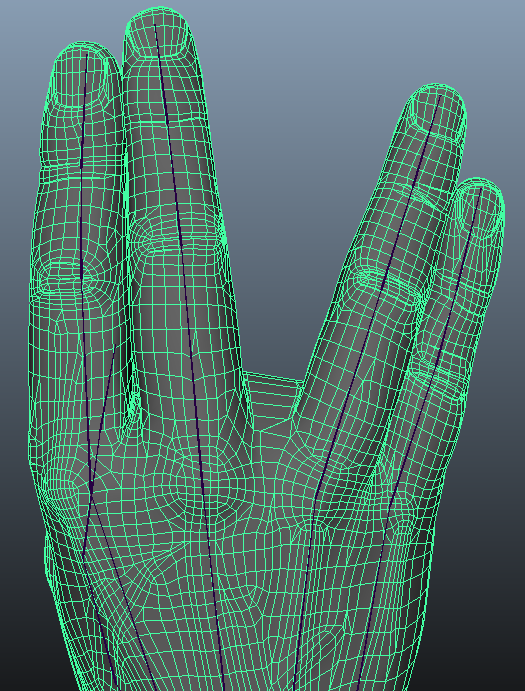# Bulge free Dual Quaternion Skinning (Trick)Left: standard DQS. Right DQS bulge correction (both use same automatic skin weight: "smooth bind" inside Maya).

I provide a C++ code snippet to correct the Dual Quaternion Skinning bulge artifact. This code reproduces the article "Bulging-free dual quaternion skinning"  from Kim, YoungBeom and Han. This trick is fast and easy to implement, however, in specific cases it will produce visible discontinuities in the final mesh deformation.

Here is how the trick works, first we compute (and possibly store) the distance of vertices from their nearest bone segment. While animating, we detect vertices that have got farther away from their bone segment, then we project back towards the bone segment to maintain the original distance. The nearest bone is determine through skinning weights. Note that by construction vertices only bulge around the outside of a bended joint, therefore, only those vertices are altered.

This re-projection trick may introduce visible discontinuity in the mesh deformation and hence in the texture or light reflection. While most joints does not exhibit this problem, especially with coarse mesh, some configuration are more prone to this artifact. On a hand model problems may arise when spreading the fingers. In areas in between the knuckles, neighboring vertices may be projected in opposite directions. This problem is also encountered in the crotch area when doing the split. Left no bulge correction, right bulge correction enabled:struct Dqs_fix {
// A bone is defined as a segment (origin, direction and length in 3D space)
std::vector<anim::Bone> _bone_rest_pose;
// _weights[vertex Index] == map<bone_index, bone_skinning_weight>
std::vector< std::map<int, float> > _weights;

/// Bulging-free dual quaternion skinning
/// "YoungBeom Kim andJungHyun Han"
inline Vec3 apply(
int id, // Index of the vertex we correct
const Point3& rest_vert, // Vertex position at bind pose / T pose
const Point3& dq_in, // Vertex after dual quaternion skinning
const std::vector<Mat4x4> skinning_transfo) // Global transformation for each joint
{
// find the index of the bone with the highest weight for vertex id
int major_bone = anim::find_max_index(_weights[id]);

// Find out the distance to the bone in rest pose and after skinning.
const anim::Bone& bone_rest = _bone_rest_pose[major_bone];
float dv_rest = bone_rest.dist_from_segment(rest_vert);

const anim::Bone bone_current = bone_rest.transform(skinning_transfo[major_bone]);
float dv_current = bone_current.dist_from_segment(dq_in);

Vec3 result = dq_in;

// If dv has grown then bulging is detected so we draw the vertex towards
// the closest bone point.
if (dv_current > dv_rest)
{
Point3 proj_point = bone_current.project_on_segment(dq_in);
Vec3 proj_dir  = dq_in - proj_point;
const float  factor = dv_rest / dv_current;
result = proj_point + (factor * proj_dir);
}
return result;
}

};

// -------------------------------------------------------------------------

/// Finds the max index in a weight map.
int find_max_index( const std::map<int, float> >& weights)
{
auto it = weights.begin();
auto maxit = weights.begin();
float max = 0.f;
for( ;  it != weights.end(); ++it) {
if (it->second > max)
{
max = it->second;
maxit = it;
}
}
assert(maxit->second == max);
return maxit->first;
}

// -------------------------------------------------------------------------

float Bone::dist_from_segment(const Point3& p) const {
Vec3 op = p - _org;
float x = op.dot(_dir) / (_length * _length);
x = fminf(1.f, fmaxf(0.f, x));
Point3 proj = _org + _dir * x;
float d = proj.distance_squared(p);
return sqrtf(d);
}

// -------------------------------------------------------------------------
Point3 Bone::project_on_segment(const Point3& p) const
{
const Vec3 op = p - _org;
float d = op.dot(_dir.normalized()); // projected dist from origin

if(d < 0)            return _org;
else if(d > _length) return _org + _dir;
else                 return _org + _dir.normalized() * d;
}

## Reference:

Alternative approaches (update)

One comment